• ## 详解 误差反向传播算法推导

万次阅读 多人点赞 2018-09-14 03:58:31
误差反向传播算法误差 反向传播算法（back propagation，简称BP模型）是1986年由Rumelhart和McClelland为首的科学家提出的概念，是一种按照误差逆向传播算法训练的多层前馈神经网络，是目前应用最广泛的神经网络...
误差反向传播算法误差
反向传播算法（back propagation，简称BP模型）是1986年由Rumelhart和McClelland为首的科学家提出的概念，是一种按照误差逆向传播算法训练的多层前馈神经网络，是目前应用最广泛的神经网络。
误差反向传播算法系统的解决了多层神经网络隐含层连接权学习问题，人们把采用这种算法进行误差校正的多层前馈网络称为BP网。BP神经网络具有任意复杂的模式分类能力和优良的多维函数映射能力，解决了简单感知器不能解决的异或(Exclusive OR，XOR)和一些其他问题。
从结构上讲，BP网络具有输入层、隐藏层和输出层；
从本质上讲，BP算法就是以网络误差平方为目标函数、采用梯度下降法来计算目标函数的最小值。
因此学习误差反向传播算法对于深度学习的深造起到非常重要的作用，这也是本篇博客的主题。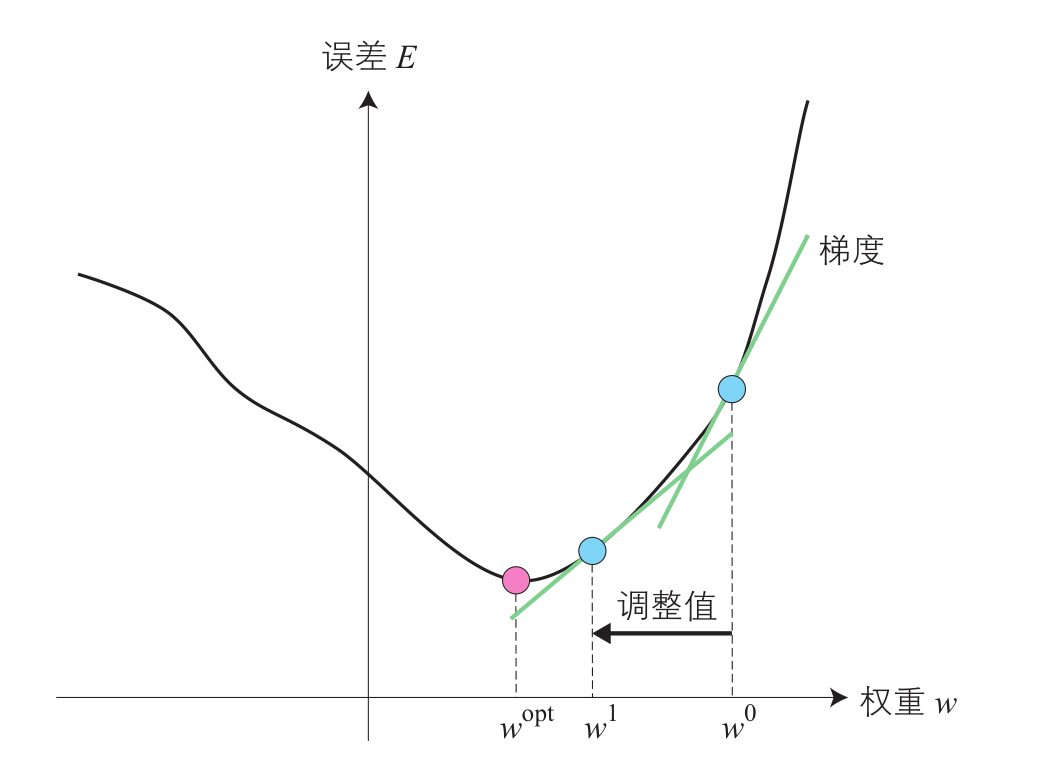由于梯度下降法需要求解相邻层的梯度，这就要求网络中需要处处可导，也就是需要激活函数也必须支持可导性。M-P模型中使用step函数作为激活函数，只能输出0或1，不连续所以不可导。为了使误差能够顺利传播，科学家们提出了可导函数sigmoid作为激活函数$f(u)$，但是在后期的使用中发现sigmoid函数也存在一定的问题（梯度饱和问题），于是发展出了ReLU函数及其变形激活函数，想深入了解激活函数的小伙伴可以查看下这篇博客：https://blog.csdn.net/ViatorSun/article/details/82418578。
1） 以单层感知器入
反向传播算法便于大家理解，下面先解释下单层感知器的梯度下降法。由复合函数求导法则可以知道，误差函数求导如下所示：
$\frac{\partial E}{\partial w_i} = \frac{\partial E}{\partial y}\frac{\partial y}{\partial w_i}$
设$y=f(u)$，求误差函数 $E$ 对 $w_i$ 的导数为：
$\frac{\partial E}{\partial w_i} = -(r-y)\frac{\partial y}{\partial w_i} = -(r-y)\frac{\partial f(u)}{\partial w_i}$
$f(u)$的导数就是对复合函数求导
$\frac{\partial E}{\partial w_i} = -(r-y)\frac{\partial f(u)}{\partial u} \frac{\partial u}{\partial w_i}$
$u$对$w_i$求导的结果只和$x_i$相关：$\frac{\partial u}{\partial w_i} = x_i$ 整理下上面两个式子，得到：
$\frac{\partial E}{\partial w_i} = -(r-y)x_i\frac{\partial f(u)}{\partial u}$
在此，我们对激活函数$Sigmoid$  ： $\sigma(x) = \frac {1}{1+e^{-x}}$函数求导：
$\frac{\partial \sigma(x)}{\partial x} = \frac {e^{-x}}{(1+e^{-x})^2}$
令 $e^{-x} = u$ 则导函数为：
$\frac{\partial f(u)}{\partial u} = f(u)(1-f(u))$
将激活函数的导数代入上面整理结果得到：
$\frac{\partial E}{\partial w_i} = -(r-y)x_i f(u)(1-f(u))$
由于输出结果$y=f(u)$，所以单层感知器的权重调整值为（其中$\eta$为学习率）：
$\Delta w_i = - \eta\frac{\partial E}{\partial w_i} = \eta(r-y)y(1-y)x_i$
至此，这就是单层感知器权重的调节量。

2）多层感知器的反传传播算法
接下来，我们再分析下多层感知器。多层感知器的误差函数$E$等于个输出单元的误差总和。$E = \frac{1}{2} \sum_{j=1}^q (r_j - y_j)^2$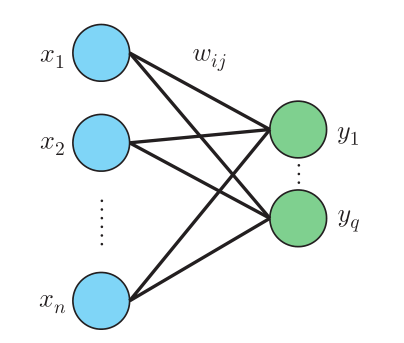对误差函数求导得：
$\frac{\partial E}{\partial w_{ij}} = \frac{\partial E}{\partial y_j} \frac{\partial y_j}{\partial w_{ij}}$
其中，$w_{ij}$代表$x_i$和$y_j$之间的连接权重，对$E$求导的结果只和$y_j$相关，如下所示：
$\frac{\partial E}{\partial w_{ij}} = -(r_j - y_j) \frac{\partial y_j}{\partial w_{ij}}$
与单层感知相同，对上式展开后对复合函数求导：
$\frac{\partial E}{\partial w_{ij}} = -(r_j - y_j) \frac{\partial y_j}{\partial u_j} \frac{\partial u_j}{\partial w_{ij}}$
下面与单层感知器一样，对误差函数求导得：
$\frac{\partial E}{\partial w_{ij}} = -(r_j - y_j) y_j (1-y_j)x_i$
则权重的调节值为（其中$\eta$为学习率）：
$\Delta w_{ij} = \eta(r_j - y_j )y_j (1-y_j)x_i$
由此可见，多层感知器中，只需要使用与连接权重$w_{ij}$相关的输入$x_i$和输出$y_j$，即可计算出连接权重的调节值。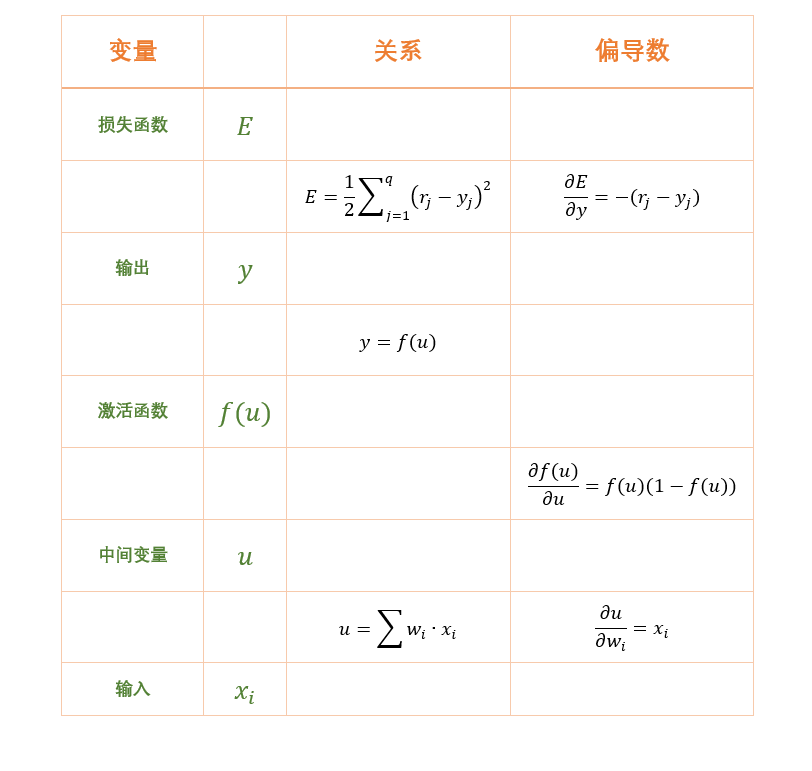将神经网络分解开可以更清晰的分析，再将最后一列各变量之间的偏导数累成就是整个链式法则的体现。

3） 带中间层的多层感知器的反向传播算法
最后我们再解释下带中间层的多层感知器的梯度下降法。由于中间层的加入，层之间的权重下标我们增加到三个，其中$i$表示输入层单元，$j$表示中间层单元，$k$表示输出层单元   。如下图所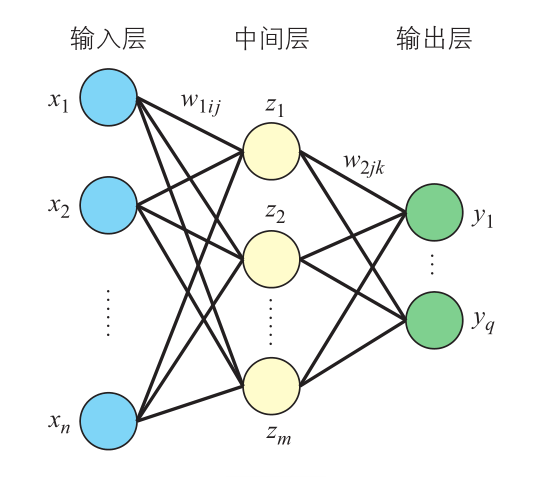首先考虑输出层与中间层之间的连接权重$w_{2jk}$的调整。对权重$w_{2jk}$求导：
$\frac{\partial E}{\partial w_{2jk}} = \frac{\partial E}{\partial y_k} \frac{\partial y_k}{\partial u_{2k}} \frac{\partial u_{2k}}{\partial w_{2jk}}$
经过误差函数$E$对输出$y_k$求导，输出$y_k$求导，输出$y_k$对激活值$u_{2k}$求导，激活值$u_{2k}$对连接权重$w_{2jk}$求导之后，得到：
$\frac{\partial E}{\partial w_{2jk}} = -(r_k - y_k) y_k ( 1-y_k) z_j$
所以，即便是输出层有多个单元，只要对每个输出单元分别求导后，也能得到误差函数对中间层权重的偏导数接下来计算输入层和中间层之间的连接权重$w_{1ij}$的偏导数：
$\frac{\partial E}{\partial w_{1ij}} = \sum_{k=1}^q[ \frac{\partial E}{\partial y_k} \frac{\partial y_k}{\partial u_{2k}} \frac{\partial u_{2k}}{\partial w_{1ij}}]$
中间层的单元$j$和输出层的所有单元相连，所以如上式所示，误差函数$E$对连接权重$W_{1ij}$求偏导，就是对所有输出单元的导数进行加权和，实际使用的是所有输出单元连接权重的总和。将$sigmoid$函数的导数和误差函数代入到上式，得：
$\frac{\partial E}{\partial w_{1ij}} = -\sum_{k=1}^q[ (r_k - y_k)y_k(1-y_k) \frac{\partial u_{2k}}{\partial w_{1ij}}]$
由于连接权重$w_{1ij}$只对中间层$z_j$的状态产生影响，所以上式中剩余部分求导后的结果如下：
$\frac{\partial u_{2k}}{\partial w_{1ij}} = \frac{\partial u_{2k}}{\partial z_j } \frac{\partial z_j}{\partial w_{1ij}}$
激活值$u_{2k}$对$z_j$求导得到连接权重$w_{2jk}$，结合下式就可以求出输入层与中间层之间的连接权重$w_{1ij}$的调整值：
$\frac{\partial z_j}{\partial w_{1ij}} = \frac{\partial z_j}{\partial u_{1j}} \frac{\partial u_{1j}}{\partial w_{1ij}} = z_j ( 1- z_j) x_i$   $\Delta w_{1ij} = \eta \sum_{k=1}^q [ (r_k - y_k) y_k ( 1- y_k) w_{2jk} ] z_j (1-z_j) x_i$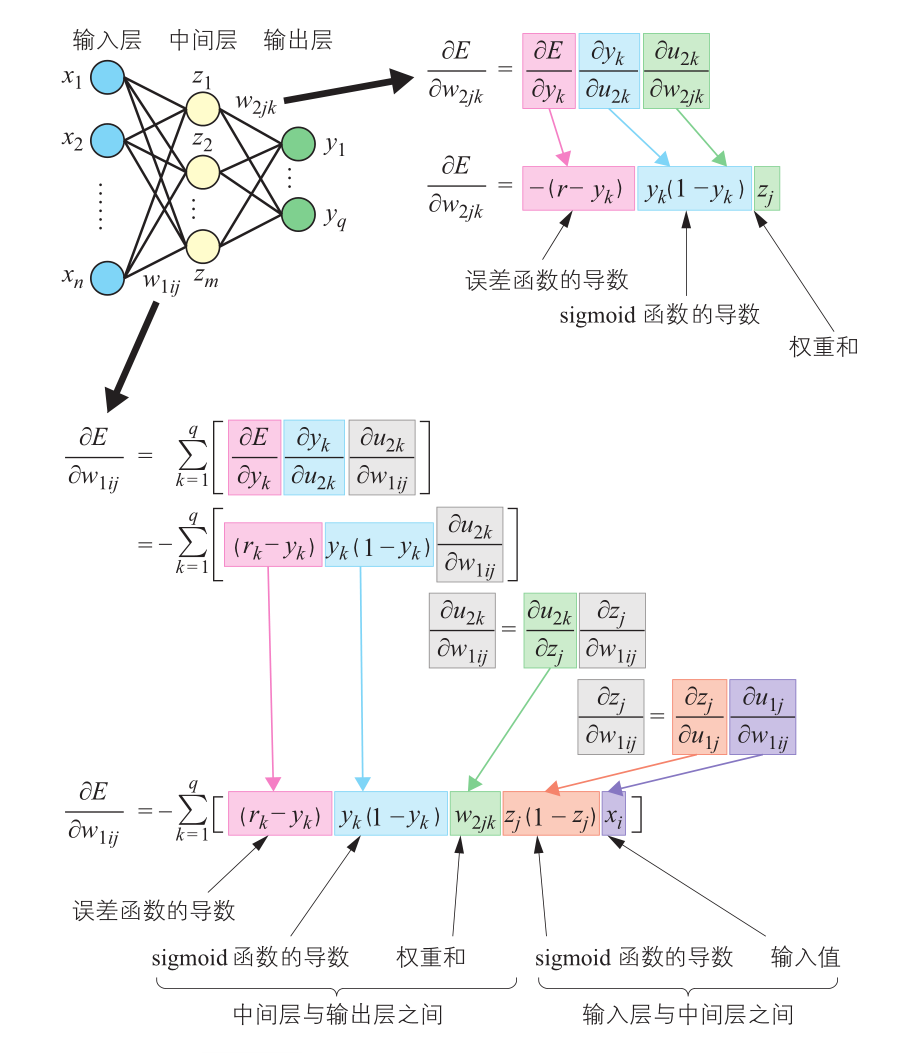具体推导过程可以参考下列公式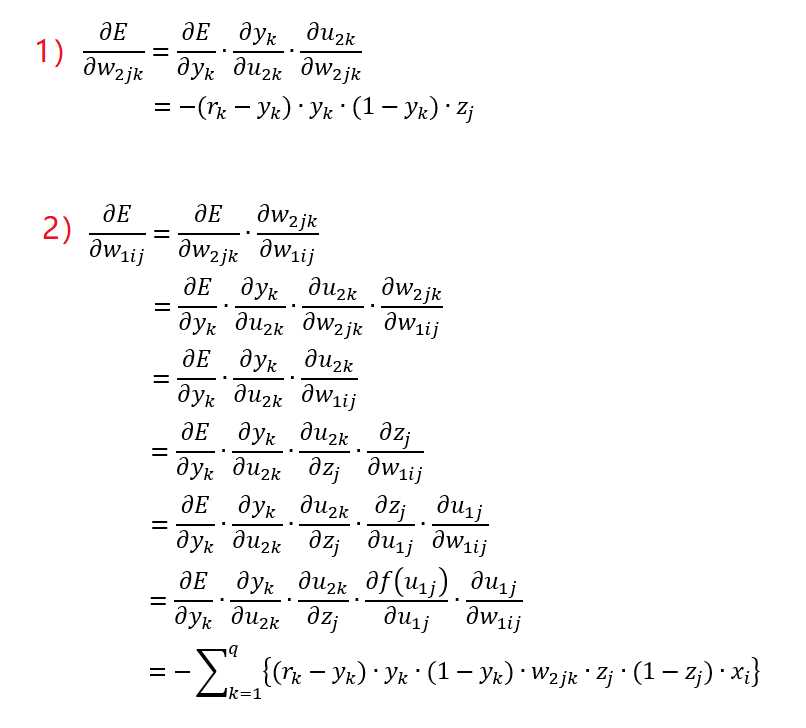在此需要注意$z_j=f(u_j)$，输入层与中间层之间的权重调整值是相关单元在中间层与输出层之间的权重调整值的总和。
4）小结
至此，误差反向传播算法的讲解就全部结束了，其中包含了大量的公式，理解起来可能会有一些难度，但是这是必过的槛。如果实在不理解过程的话，只记住最后那张图也可以，那张图便是整个算法的精髓所在。除此之外，在实际应用过程中可能还会遇到一个问题，那就是激活函数使用$Sigmoid$或者$tanh$函数的时候，如果$x$趋向正负无穷的时候，会出现偏导数为零的情况，见下图，左侧为$Sigmoid$函数图像，右侧为其导函数的图像。这时候，由于权重调整值趋近于0，所以无法调整连接权重，权重所在的单元也就不再起作用。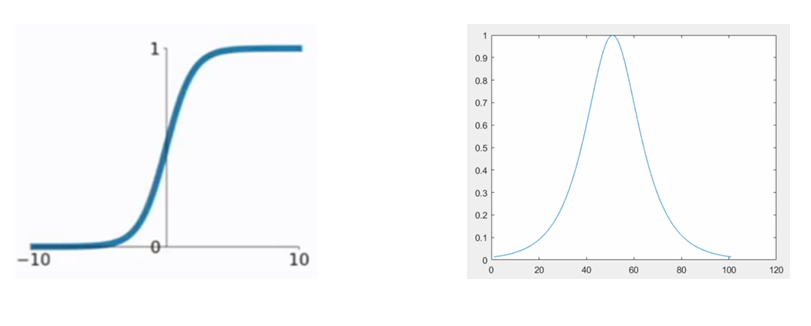这就是误差反向传播算法中的梯度消失导致无法调整连接权重的问题，对于这个问题，需要在训练的过程中合理地调整学习率$\eta$，以防止梯度消失。展开全文BP网络
• 反向传播（英语：Backpropagation，缩写为BP）是“误差反向传播”的简称。由于多层前馈神经网络的训练经常采用误差反向传播算法，人们也常把多层前馈神经网络称为BP网络。

反向传播（英语：Backpropagation，缩写为BP）是“误差反向传播”的简称。由于多层前馈神经网络的训练经常采用误差反向传播算法，人们也常把多层前馈神经网络称为BP网络。

反向传播算法发明的历史请参考我的前文《神经网络简史》。

多层前馈神经网络是指通过按某种方式将神经元连接起来，就可构成相关神经网络。下图所示为一个熟知的前馈网络模型，该网络有L层，第1 层为输入层，第L层为网络输出层。在这个网络中，前一层神经元全连接到后一层神经元，同层的神经元之间没有任何连接。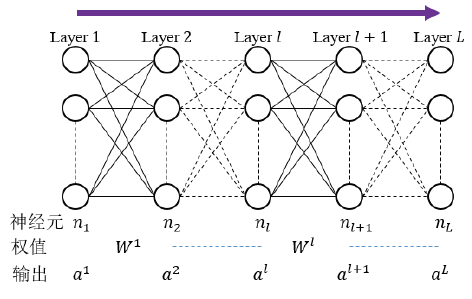反向传播通常被认为是基于优化理论的一种监督式学习方法，虽然它也用在一些无监督网络（如自动编码器）中。

本文主要内容：

神经网络应用梯度下降的直观理解

反向传播算法的学习过程

反向传播算法推导

总结探讨

本文灵感来源

参考文献

直观理解

考虑一个有两个输入单元、一个输出单元、没有隐藏单元的简单神经网络。每个神经元都使用输入的加权和作为线性输出。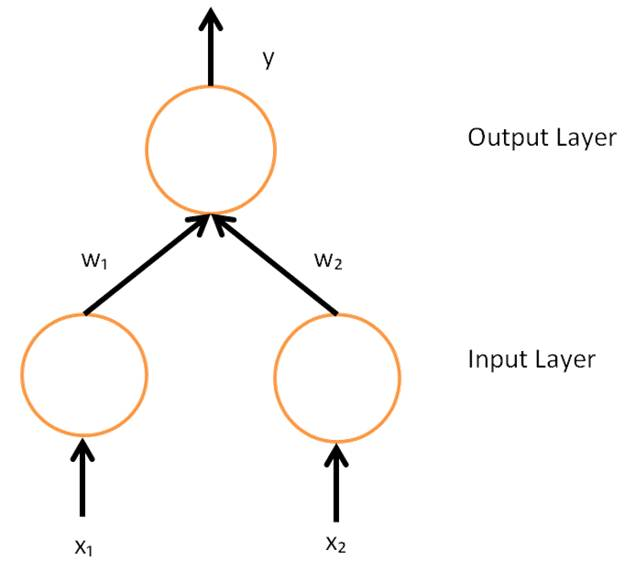最初在训练之前，会随机分配权重。之后神经元根据训练实例进行学习，在此情况下包含元组 (x1,x2,t) 的集合，其中 x1与x2是网络的输入，t 为正确输出（在给定相同的输入时网络最终应当产生的输出）。网络在给定x1 和 x2时，会计算一个输出 y，很可能与t 不同（因为权重最初是随机的）。衡量期望输出t 与实际输出  y 之间的差异的一个常见方法是采用平方误差测度：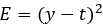其中E为差异或误差。

为什么采用平方差？其数学背景是最小二乘法，也可以理解为空间两点的距离或者平方误差等。最小二乘法（又称最小平方法）是一种数学优化技术。它通过最小化误差的平方和寻找数据的最佳函数匹配。最重要的应用是在曲线拟合上。最小平方所涵义的最佳拟合，即残差（残差为：观测值与模型提供的拟合值之间的差距）平方总和的最小化。

举例来讲，考虑单一训练实例的网络：(1,1,0)，输入x1 与x2均为1，正确输出t 为 0（网络只有一个输出）。现在若将实际输出 y 画在x轴，误差E画在y 轴，得出的是一条抛物线。抛物线的极小值对应输出y，最小化了误差E。对于单一训练实例，极小值还会接触到
x 轴，这意味着误差为零，网络可以产生与期望输出t 完全匹配的输出y。因此，把输入映射到输出的问题就化为了一个找到一个能产生最小误差的函数的最优化问题。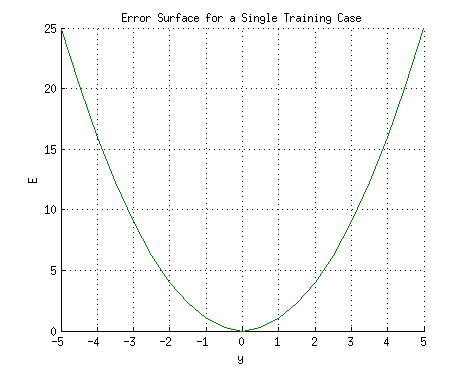单一实例的神经网络的误差函数非常容易理解，可以通过解方程，直接找到最小值。这里给一个梯度表示，如下图，便于理解多实例神经网络的梯度表示。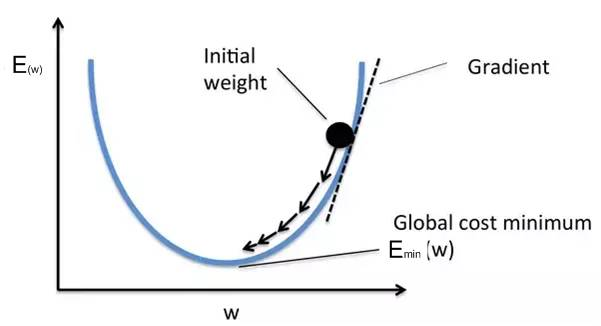反向传播算法的目的是找到一组能最大限度地减小误差的权重。寻找抛物线或任意维度中任何函数的极大值的方法有若干种。其中一种方法是通过求解方程组，但这依赖于网络是一个线性系统，而目标也需要可以训练多层非线性网络（因为多层线性网络与单层网络等价）。

如果考虑两个实例呢（依然是单层神经网络，不考虑非线性变换）？

考虑一个神经元的输出取决于其所有输入的加权总和：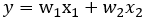其中w1和 w2是从输入单元到输出单元相连的权重。因此，误差取决于输入到该神经元的权重，也是网络要学习最终需要改变的。若每个权重都画在一个水平的轴上，而误差画在垂直轴上，得出的就是一个抛物面（若一个神经元有 k 个权重，则误差曲面的维度就会是k+1，因而就是二维抛物线的k+1维等价）。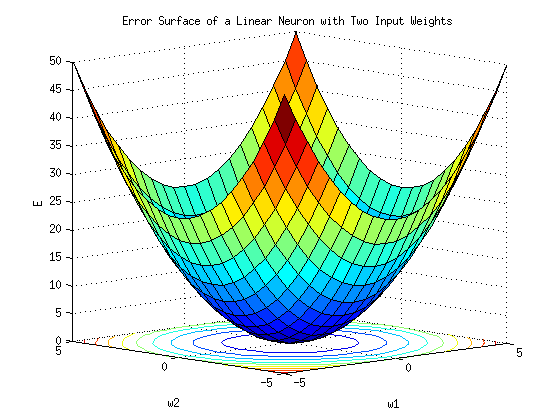抛物面的最小值需要通过梯度下降法求得。如下图所示。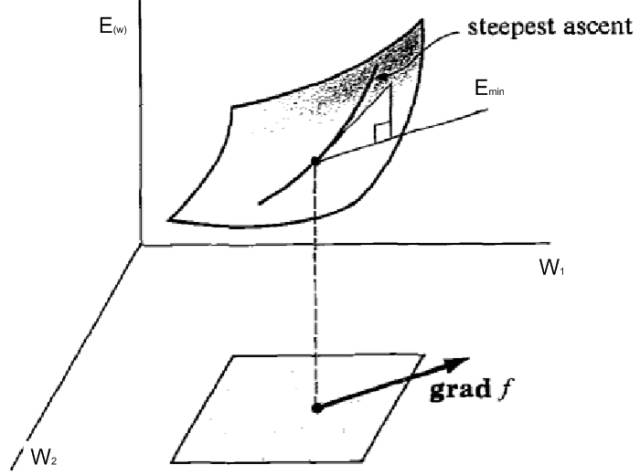如果是多个实例呢？并且是多层神经网络的非线性变换呢？从数学角度看，已经不能通过求解方程组得到最小值，也不能简单的描绘多维权重系数构成的函数所对应的几何形状（比如抛物面）。但是运用抽象推理，大概想象成这样子：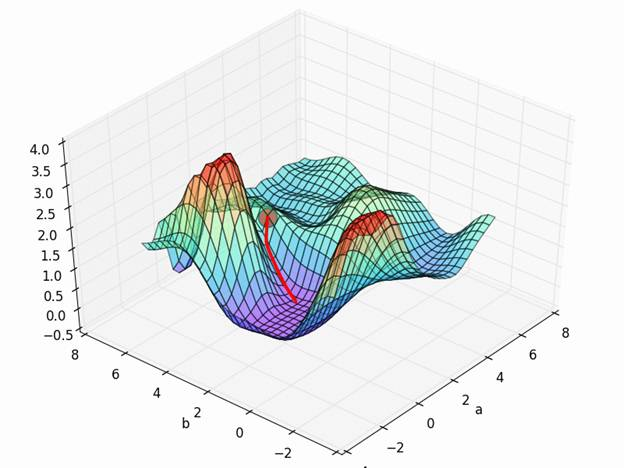以上就是神经网络学习和误差函数原理的直观表示。

结合梯度的概念，这里先给出梯度下降的推导结果（公式所示），下面逐步解释为什么有这个推导结果。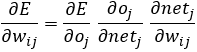这个公式推导所带出的三个导数非常重要，是影响梯度的三个重要因素。我把上面三个偏导分成三部分来讨论，分别对应于误差函数，激活函数，神经元模型。假设我们要得到的是第i层到第j层的连接权重w_ij，用于梯度的偏导可以通俗的表达为：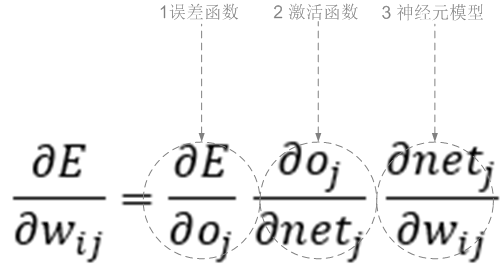下面分别从神经元模型，误差函数和激活函数的角度解释这个公式。

一个神经元的基本模型如下图所示。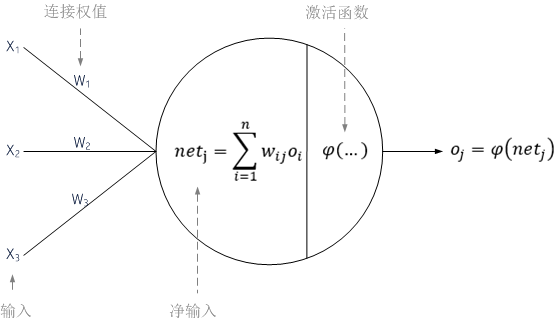通向一个神经元的输入net_j是之前神经元的输出o_i 的加权和。若该神经元输出层后的第一层，输入层的输出 o_i就是网络的输入 x_i。该神经元的输入数量是 n。变量 w_ij表示神经元 i 与 j 之间的权重。对于每一个神经元，其对应的输出为：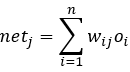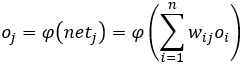从数学的角度来看，神经元模型定义了两层的复合函数：内层是net_k，外层是φ函数。激活函数φ一般是非线性可微函数（反向传播要求人工神经元的激励函数可微）。常用作激活函数的是Sigmoid函数：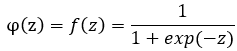或者写成：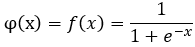这个函数也被称为单极性Sigmoid函数。其导数形式很好：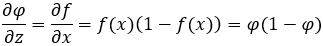其图像如何下：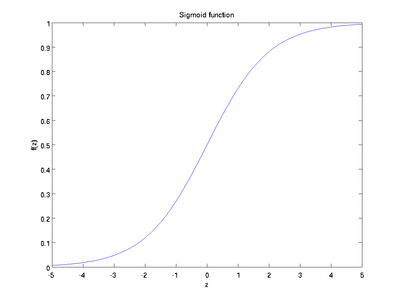双极性Sigmoid函数要比较常用。公式如下：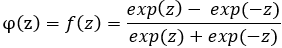或者写成（把上式中分子分母同时除以e^z，令x=-2z就得到第二个式子）：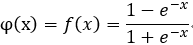其导数为：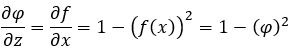其图像为：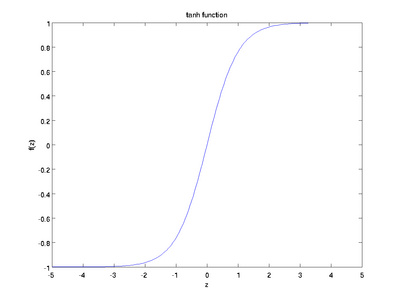从上图可以看出，对于Sigmoid函数，当z的取值越来越大后（饱和区），函数曲线变得越来越平缓，意味着此时的导数也越来越小。同样的，当z的取值越来越小时（饱和区），也有这个问题。仅仅在z取值为0附近时，导数的取值较大。在后文讲到的反向传播算法中，每一层向前递推都要乘以导数，得到梯度变化值。Sigmoid的这个曲线意味着在大多数时候，我们的梯度变化值很小，导致我们的W,b更新到极值的速度较慢，也就是我们的算法收敛速度较慢。

由于反向传播使用梯度下降法，需要计算平方误差函数对网络权重的导数。假设对于一个输出神经元，平方误差函数为：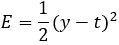其中

E 为平方误差，

t 为训练样本的目标输出，

y 为输出神经元的实际输出。

加入系数1/2是为了抵消微分出来的指数。之后，该表达式会乘以一个任意的学习速率，因此在这里乘上一个常系数是没有关系的。

由梯度的定义，与方向导数有关联的一个概念是函数的梯度。多元函数的偏导向量构成了梯度，梯度的方向是函数在这点增长最快的方向，那么函数的偏导在这点的梯度方向也达到最大值。其中，要使用梯度下降法更新 w_ij，必须选择一个学习速率μ。要加在原本的权重上的变化，等于学习速率与梯度的乘积，乘以-1：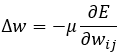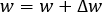之所以要乘以-1 是因为要更新误差函数极小值而不是极大值的方向。

从数学的角度看，平方误差函数形成了对输出o_j的复合函数：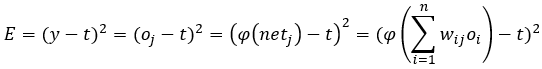这个式子无论对于输出层的神经元，还是隐藏层的神经元，都是成立的。

综上所述，误差函数是关于权重的函数，为了在由权重系数构成的多维空间中寻找一个下降最快的梯度方向，我们需要对所有权重系数求偏导。根据复合函数的求导规则，其一般形式为：反向传播算法的学习过程

学习过程由信号的正向传播与误差的反向传播两个过程组成。正向传播时，输入样本从输入层传入，经各隐层逐层处理后，传向输出层。若输出层的实际输出与期望的输出(教师信号) 不符，则转入误差的反向传播阶段。误差反传是将输出误差以某种形式通过隐层向输入层逐层反传，并将误差分摊给各层的所有单元，从而获得各层单元的误差信号，此误差信号作为修正各单元权值的依据。这种信号正向传播与误差反向传播的各层权值调整过程，是周而复始地进行的。权值不断调整的过程，也就是网络学习训练过程。此过程一直进行到网络输出的误差减少到可接受的程度，或进行到预先设定的学习次数为止。

学习过程的伪码描述如下：

输入：训练集和学习率

初始化网络权值（通常是小的随机值）

do

forEach 训练样本 ex

prediction = neural-net-output(network, ex)  // 正向传递，得到当前样本的输出

actual = teacher-output(ex)//从监督老师那里获得真实输出

计算输出单元的误差 (prediction - actual)//获得残差

计算    对于所有隐藏层到输出层的权值和阈值          // 反向传递

计算    对于所有输入层到隐藏层的权值和阈值          // 继续反向传递

更新网络权值和阈值 // 输入层不会被误差估计改变

until 所有样本正确分类或满足其他停止条件

return 权重与阈值确定的多层前馈神经网络

完整的误差反向传播算法包括前向计算和反向传播两部分。

反向传播算法推导

为了最小化误差E，最终归结为优化问题。前面说过，反向传播算法的目的是找到一组能最大限度地减小误差的权重，在反向传播中使用的方法是梯度下降法。这样我们就需要计算误差函数E对权重的偏导。由上文，误差函数对权重w_ij的偏导数是三个偏导数的乘积：我们的目标就是分别求得这三个偏导。

在右边的最后一项中（神经元模型），只有加权和 net_j取决于 w_ij，因此：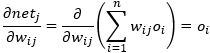当对一个权重求导时，其他权重就视为常量。这里如有不确定，把加权和展开即可明白。

对于激活函数部分，神经元j 的输出对其输入的导数就是激活函数的偏导数（这里假定使用Sigmoid函数）：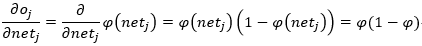这就是为什么反向传播需要的激活函数是可微的。同时，前向传播时，很容易求得net_j（各层神经元计算得到的加权和），所以该偏导也容易求得。

对于误差函数部分的偏导，为了方便理解，分输出层和隐藏层来讨论。

如果神经元在输出层中，因为此时o_j=y以及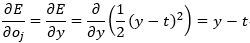所以第一项可以直接算出。

但如果j 是网络中任一内层（隐藏层），就需要用到链式求导法则。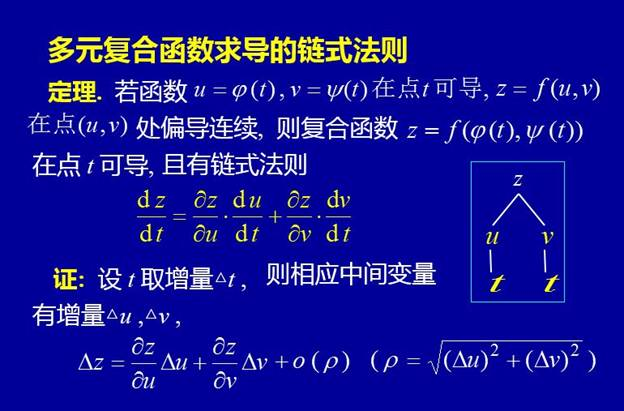下面以一个神经网络的局部来说明。神经网络中相邻的两层构成一个计算单元，因此只需要理解第j层和第k(j+1) 层之间的结构和运算，便可以通晓整个网络的结构和运算（前面说过，层与层之间全连接，同层之间没有连接）。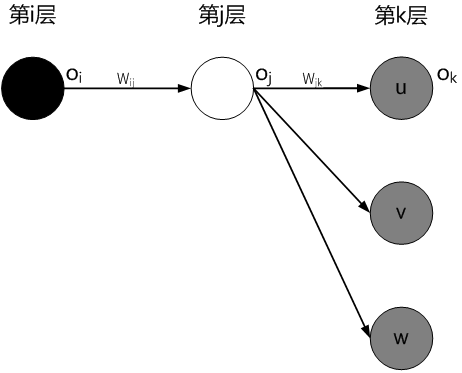注意到我们要求的是第i层到第j层的连接权重w_ij，考虑第j层中的某个神经元的输出o_j是第k层所有神经元{u,v,…,w}的输入，把误差（损失）函数E看作是{u,v,…,w}的函数（注意，这里的第k层可能是输出层，也可能是隐藏层；作为输出层时，既可能是多值输出，也可能是单值输出）。可以得到：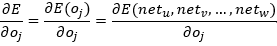这里应用链式求导法则：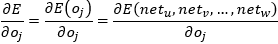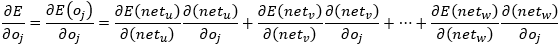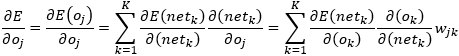最后一步做了两次替换：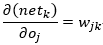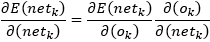对于上面推导结果，我们发现：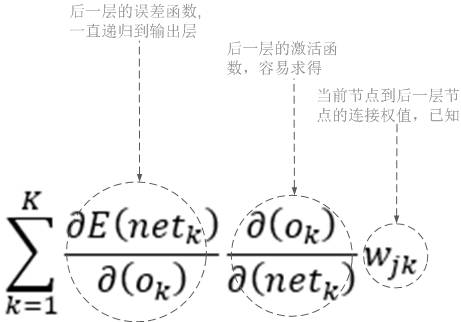因此，若已知所有关于下一层（更接近输出神经元的一层）的输出关于o_k 的导数，则可以计算 o_j的导数。

现在把上述推导放在一起：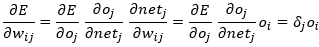此时：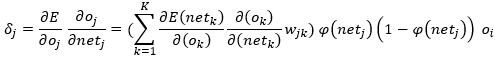则：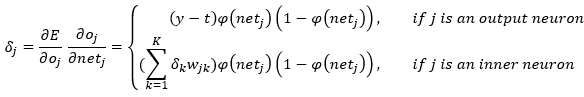综上，权重的调整只和以下因素有关：

前向神经元的输出（和所调整权重有关的一个前向神经元的输出作为输入）

当前神经元的激活函数

所有后向神经元（误差函数导数，激活函数导数，并一直递归下去）及其前向传播时的权重（这些权重用来作为误差后向传播的权重）

递归会终结在输出层，从而使用残差(y-t)。注意到递归的层数以及系数作用，这里有一大串连乘，如果连乘的数字小于1，则梯度越乘越小，导致梯度消散；如果连乘的数字大于1，则梯度越乘越大，导致梯度爆炸

对于偏置来说，注意到偏置是没有权重，调整的是偏置本身

总结探讨

总结起来，BP的误差反向传播思想可以概括为：利用输出层的误差来估计出其直接前导层的误差，再借助于这个新的误差来计算更前一层的误差，按照这样的方式逐层反传下去便可以得到所有各层的误差估计。

BP算法的提出在一定程度上解决了多层网络参数训练难的问题，但是其自身也存在如下一些问题。

首先，误差在反向传播过程中会逐渐衰减/增大，经过多层的传递后将会变得消散/爆炸，这使得BP在深层网络中并不可行。对于梯度爆炸，则一般可以通过调整神经网络模型中的初始化参数得以解决。对于无法完美解决的梯度消失问题，目前有很多研究，一个可能部分解决梯度消失问题的办法是使用ReLU（Rectified Linear Unit）激活函数（σ(z)=max(0,z)）。

其次，BP采用最速梯度下降的优化思想，而实际问题的误差函数通常不是凸的，存在众多局部极小值点，算法很难得到最优解。极小值问题，有多种解决方案，比如从多个不同的初始点开始搜索，模拟退火，随机梯度下降，遗传算法等。但这些都是启发式，理论上尚缺乏保障。

第三，由于训练过程中依靠于导数信息来进行权值的调整，当权值调节过大时会使大部分神经元的加权和过大，致使传递函数工作于Ｓ型函数的饱和区，所以权值的调整会出现停顿的情况。

第四，隐层神经元的个数设置是个未解决的问题，实际应用中通常靠试错法调整。

第五，BP神经网络的过拟合，常用的方法是早停和正则化。早停是指：将数据分成训练集和验证集，训练集用来计算梯度，更新连接权和阈值，验证集用来估计误差，如果训练集误差降低但是验证集误差升高，则停止训练，同时返回具有最小验证集误差的连接权和阈值。正则化是指：误差目标函数中增加一个用于描述网络复杂度的部分，例如连接权与阈值的平方和。

最后，对于一些复杂网络的优化问题，BP算法受到学习速率的限制需要花费几个小时，甚至更长的时间来完成训练任务。累积BP算法和标准BP算法各有优缺点。

展开全文• 误差反向传播算法 我们知道神经网络是由大量的参数即权重构成，神经网络的学习过程则是这些参数的变化更新过程。误差反向传播算法就是用于更新这些参数的。这里我们假设激活函数为Sigmoid。 Ps: 在吴恩达...
误差反向传播算法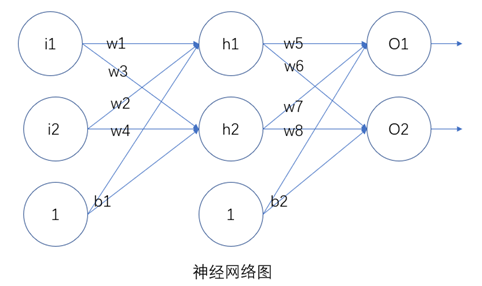我们知道神经网络是由大量的参数即权重构成，神经网络的学习过程则是这些参数的变化更新过程。误差反向传播算法就是用于更新这些参数的。这里我们假设激活函数为Sigmoid。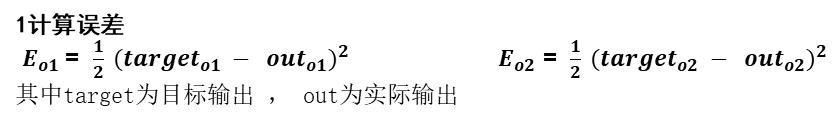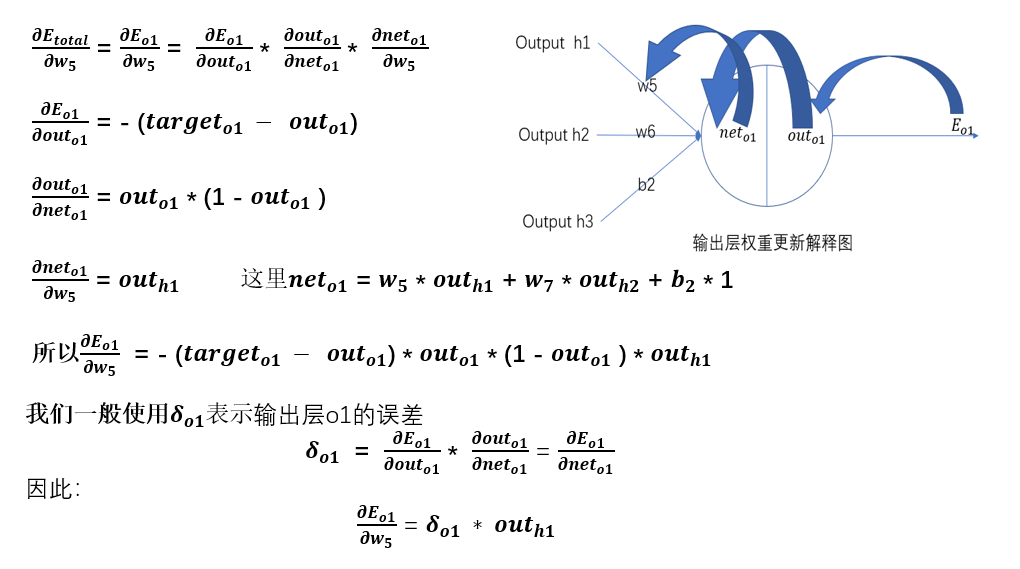Ps:  在吴恩达机器学习反向传播算法课程中，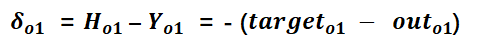而不是：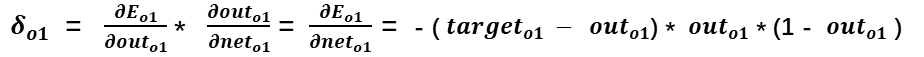这是因为吴恩达中使用的损失函数为：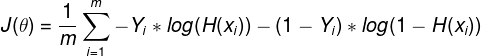因此：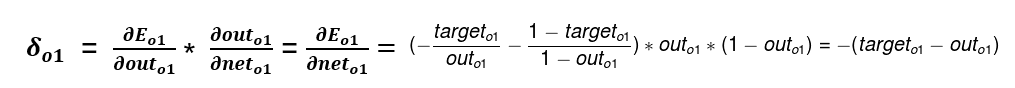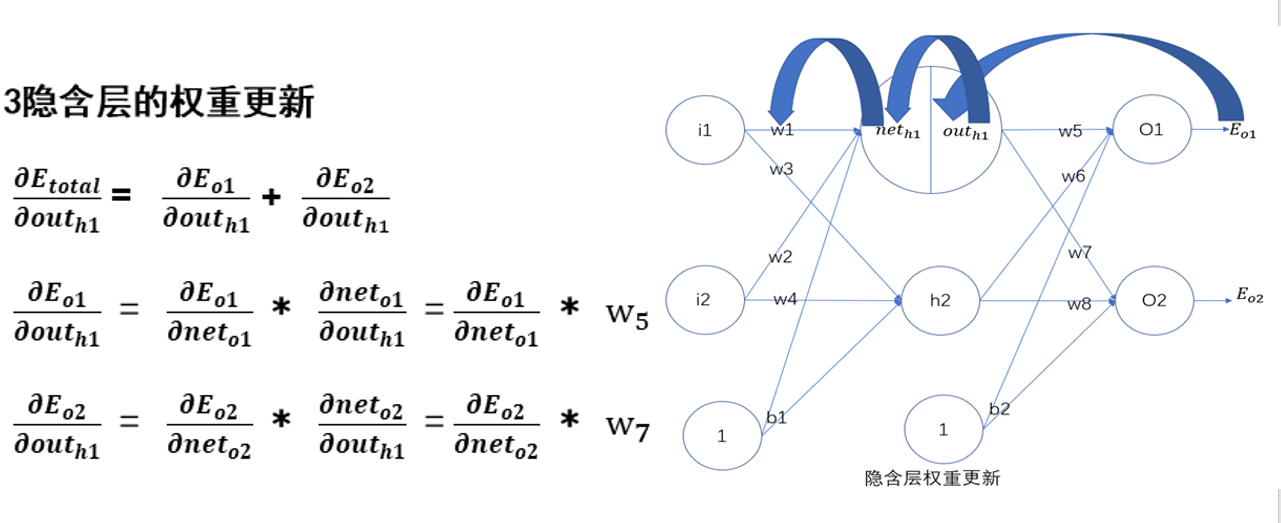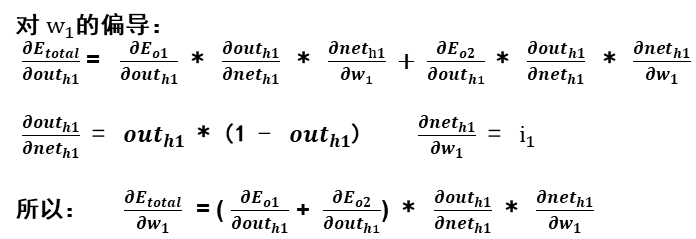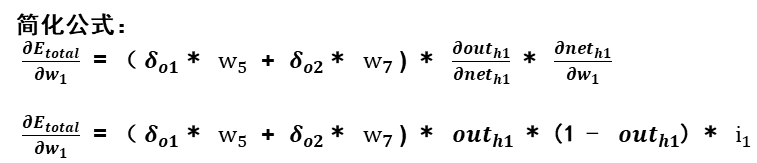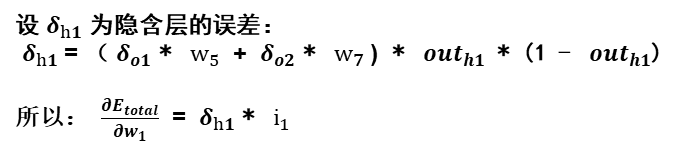展开全文机器学习
• 1.误差反向传播算法是干什么的？ 通过数值微分计算了神经网络的权重参数的梯度，数值微分虽然简单，也容易实现，但缺点是计算上比较费时间。本章我们将学习一个能够高效计算权重参数的梯度的方法——误差反向传播法...
文章目录一.误差反向传播算法是干什么的？二.计算图(一) 了解计算图的写法(二)局部计算(三) 计算图的优点三. 误差反向传播算法的具体实现

一.误差反向传播算法是干什么的？
通过数值微分计算了神经网络的权重参数的梯度，数值微分虽然简单，也容易实现，但缺点是计算上比较费时间。本章我们将学习一个能够高效计算权重参数的梯度的方法——误差反向传播法。
用梯度的方法计算神经网络的权重参数，虽然理解起来很容易理解，但是费时间。

二.计算图
(一) 了解计算图的写法计算图是从左到右的进行计算， 圆圈里面写 计算符号 ，将计算的中间结果写在箭头的上方，表示各个节点的计算结果从左向右传递。
正向传播是从计算图出发点到结束点的传播，当然也可以考虑反向的传播，这种传播称为反向传播。

(二)局部计算
计算图的特征是可以通过传递“局部计算”获得最终结果。“局部”这个词的意思是“与自己相关的某个小范围”。局部计算是指，无论全局发生了什么，都能只根据与自己相关的信息输出接下来的结果。计算图可以集中精力于局部计算。无论全局的计算有多么复杂，各个步骤所要做的就是对象节点的局部计算。虽然局部计算非常简单，但是通过传递它的计算结果，可以获得全局的复杂计算的结果。

(三) 计算图的优点

简化计算过程，让我们可以集中尽力于局部的计算
可以保留中间的计算过程，比如最后的总钱数，（这个特点，在误差反向传播算法的，可以实现结果的共享，大大的加快了计算的过程）
其实更重要的，是可以通过反向传播高效的计算导数

三. 误差反向传播算法的具体实现
下面来看，具体是如何进行计算的：如图所示，反向传播使用与正方向相反的箭头（粗线）表示。反向传播传递“局部导数”，将导数的值写在箭头的下方。在这个例子中，反向传播从右向左传递导数的值（1→1.1→2.2）。从这个结果中可，“支付金额关于苹果的价格的导数”的值是2.2。这意味着，如果苹果的价格上涨1日元，最终的支付金额会增加2.2日元。
付金额关于苹果的个数的导数”等也都可以用同样的方式算出来。并且，计算中途求得的导数的结果（中间传递的导数）可以被共享，从而可以高效地计算多个导数。综上，计算图的优点是，可以通过正向传播和反向传播高效地计算各个变量的导数值。


展开全文• 误差反向传播算法（BP） 误差反向传播算法（BP） 一、神经网络基本概念 二、神经元模型 1. 单个神经元 1> 感知器模型 2> 输入与输出 3> 激活函数 2. 单个神经元的学习 1> 识别 3. 多层神经元...人工智能 深度学习 机器学习
• 针对该问题，鲁梅尔哈特的人于1986年提出了误差反向传播算法，该算法使得神经网络再次回到人们的视野。 什么是误差反向传播算法？ 通过比较实际输出和期望输出得到的误差信号，把误差信号从输出层逐层向前传播,得到...随机梯度下降 神经网络 人工智能 深度学习
• 文章目录记录TensorFlow听课笔记一，误差反向传播算法二，链式求导法则三，隐含层有多个神经元的误差反向传播四，激活函数 一，误差反向传播算法 利用链式法则，反向传播损失函数的梯度信息， 计算出损失函数对...
• 误差反向传播算法系统解决了多层神经网络隐含层连接权学习问题，人们把采用这种算法进行误差校正的多层前馈网络称为BP网。BP神经网络具有任意复杂的模式分类能力和优良的多维函数映射能力，解决了简单感知器不能解决...神经网络 机器学习 深度学习
• 计算机视觉05：神经网络与误差反向传播算法 文章目录计算机视觉05：神经网络与误差反向传播算法1. 神经网络1. 前馈神经网络（无反馈）2. Delta学习规则3. 梯度下降4. 权重如何改变5. 示例6.其他梯度下降7.典型的机器...计算机视觉
• BP神经网络：误差反向传播算法公式推导 开端： BP算法提出 1. BP神经网络参数符号及激活函数说明 2. 网络输出误差(损失函数)定义 3. 隐藏层与输出层间的权重更新公式推导 4. 输入层与隐藏层间的权重更新...神经网络 深度学习 python 机器学习
• 我们知道，计算梯度可以使用数值微分和误差反向传播算法，但数值微分计算速度慢，其计算精度会有极小的缺失，一般较少使用。使用误差反向传播算法则可以高效计算梯度，故其在深度学习是一个非常基础的知识，也是一个...
• 误差反向传播算法 一、单层感知器 期望输出rrr和网络的实际输出yyy计算最小二乘误差函数 EEE 连接权重www 、η\etaη 表学习率 、 sigmoid\text{sigmoid}sigmoid函数作为激活函数f(u)f(u)f(u) 单层感知器：∂E∂...深度学习 神经网络
• 误差反向传播算法  之前，我们使用数值微分计算了神经网络的权重参数的梯度（严格来说，是损失函数关于权重参数的梯度）。数值微分容易实现，但是缺点在于计算上比较耗费时间。所以，在训练神经网络时，一般使用...
• 1、引言 误差反向传播网络（Error Back Propagtion，），简称为...多层感知器强调神经网络在结构上由多层组成，BP神经网络则强调网络采用误差反向传播的学习算法。大部分情况下多层感知器采用误差反向传播算法进行...人工智能 神经网络
• 误差反向传播法——能够高效计算权重参数的梯度的方法。要正确理解误差反向传播法，有两种方法: 一种是基于数学式；另一种是基于计算图(computational graph)。前者是比较常见的方法，机器学习相关的图书中多数都是...神经网络 深度学习 机器学习 人工智能
• 误差反向传播算法 首先定义神经网络中的一些重要参数 wjklw^{l}_{jk}wjkl​:表示从(l−1)th(l-1)^{\mathrm{th}}(l−1)th层的第kthk^{\mathrm{th}}kth个神经元到 lthl^{\mathrm{th}}lth层的第jthj^{\mathrm{th}}jth...神经网络 深度学习
• 【开始时间】： 【结束时间】： 【论文】：王铁林, 沈国良. 误差反向传播算法的数学推导及实现步骤. 辽阳石油化高等专科学校学报，2002.9（2）1-3. 【论文链接】： ...
• 优化算法基本上都是在反向传播算出梯度之后进行改进的，因为反向传播算法是一个递归形式，通过一层层的向后求出传播误差即可。 计算Loss函数的目的：**计算出当前神经网络建模出来的输出数据与理想数据之间的距离，...
• 在讲解误差反向传播算法之前，我们来回顾一下信号在神经网络中的流动过程。请细细体会，当输入向量$$X$$输入感知器时，第一次初始化权重向量$$W$$是随机组成的，也可以理解成我们任意设置了初始值，并和输入做点积...
• BP算法通过将输出误差反向传播，将误差分配到每个权值上，利用梯度更新对所有参数进行调整以达到输出累计误差最小 [图片来自Andrew Ng的machine learning课程] 为了简化概念，先不加上偏置单元 符号介绍 zli...机器学习 bp神经网络
• output_deltas=[0.0]*self.no # 反向传播 for k in range(self.no): error=targets[k]-self.ao[k] output_deltas[k]=dsigmod(self.ao[k])*error # 隐藏层误差 hidden_deltas=[0.0]*self.nh for j in range(self.nh)...神经网络 机器学习 python代码
• 其实就是链式法则，根据你想求导的两个值，来拆分，中间有权值、激活关系，层层递进，都是以误差为分子，要改变的权重为分母来求导。 里面涉及到的公式有： 所以，中间层与输出层之间的连接权重的调整如下： 调整...
• 本节开始深度学习的第一个算法BP算法，本打算第一个算法为单层感知器，但是感觉太简单了，不懂得找本书看看就会了，这里简要的介绍一下单层感知器： 图中可以看到，单层感知器很简单，其实本质上他就是线性分类器...
• 反向传播算法又称误差反向传播、BP算法( Backpropagation,缩写为BP）。 BP算法的学习过程由正向传播过程和反向传播过程组成。 在正向传播过程中，输入信息通过输入层经隐含层，逐层处理并传向输出层。如果在输出层得...
• BP算法学习笔记 1 学习基础–神经网络的基本原理和前向过程 ​ 如下图所示，这时一个简单的神经网络模型，通过这样一个模型我们就可以完成一些工作。例如我们输入三个数据，a1表示一个公司的银行存款，a2表示所拥有...神经网络 深度学习
• 【4】误差反向传播 输出层和隐藏层的误差传播公式可统一为： 权重增量 = -1学习步长目标函数对权重的偏导数 目标函数对权重的偏导数 = -1残差当前层的输入 残差= 当前层激励函数的导数*上层反传来的误差 上层反传来...神经网络 深度学习 人工智能 机器学习
• 实际上就是一个：梯度下降反向传播更新 如果熟知高数和懂最优化的梯度下降理论，可以直接跳到 四 一、反向传播的由来 在我们开始DL的研究之前，需要把ANN—人工神经元网络以及bp算法做一个简单解释。 输入层...深度学习 梯度下降 CNN参数更新
• ） 注意：反向传播是一种自动微分技术，是一种特殊的反向模式自动微分技术，其通过构建计算图来计算所有权重的梯度。 如下是一个两层权重神经网络的计算图示意图： ∂L∂Y^=(Y^−Y).∗f2′\frac{\partial L}{\......